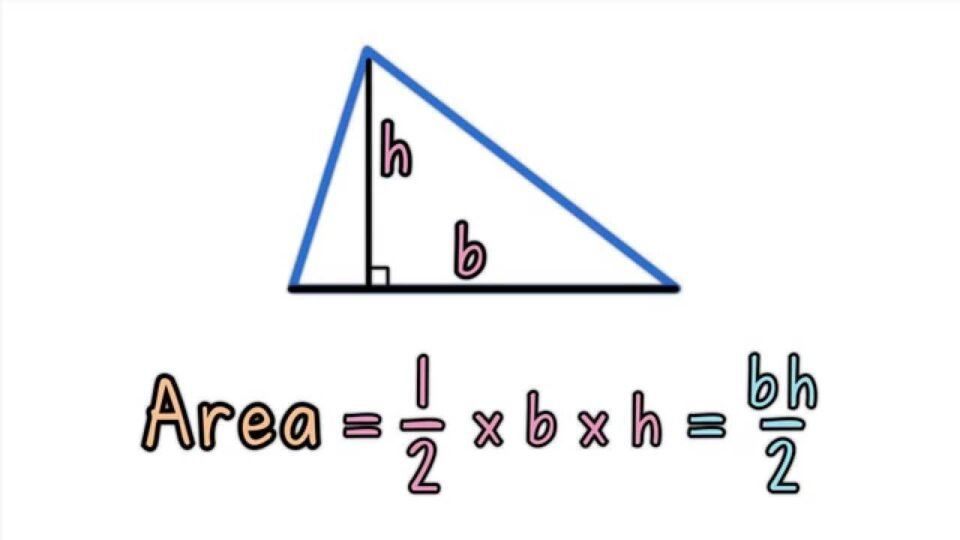Education

# Area of TriangleA polygon having three edges and three vertices are known as a triangle. In geometry, a triangle is one of the basic shapes which is two-dimensional. You might have studied the area of a triangle in your elementary school; do you remember? No! Let me tell you, the area of a triangle is a region that is enclosed within the vertices and edges of the triangle. The general formula given for the area of a triangle is half of the product multiplied by the base and height of the triangle. Mathematically, the area is equal to ½ * b*h where ‘b’ is the base and ‘ h’ is the height of the triangle. In this article, we may try to understand some related topics regarding the triangle and its area.

## Equilateral Triangle

Generally, in geometry, we define an equilateral triangle as a triangle having equal lengths that are 60 degrees. The sum of the equilateral triangle results in 180 degrees and thus it is regarded as a triangle. As mentioned above the lengths are equal in an equilateral triangle, this basically signifies that a=b=c where a, b and c are the sides of the equilateral triangle. It is also a regular polygon that contains three edges and three vertices. The perimeter of an equilateral triangle is 3a where a is the side of the respective triangle.

## Some Important Properties Of a Triangle

There are numerous properties of a triangle, but some of them are very significant and occupy most of the part in the calculations of a triangle. The following points mentioned  below analyses the properties of the triangle briefly:

1. Every triangle consists of three sides, vertices and edges respectively. A triangle is basically categorized into three types according to the lengths; an equilateral triangle, an isosceles triangle, and a scalene triangle.
2. In every triangle of any type, the sum of the angles presents internally will give the resultant value of 180 degrees. This property is popularly known as the sum of angle property.
3. One of the most significant properties of a triangle is that the value-added or the sum of two sides will always be greater than the third or the other side.
4. The largest side of a triangle will be the side opposite to the largest angle. The hypotenuse is the largest side of a triangle.

## Some Important Properties of an Equilateral Triangle

The following points mentioned below analyses some of the important properties of an equilateral triangle:

1. An equilateral triangle is a type of triangle with three sides (regular polygon) that are equal to each other in measurement.
2. An equilateral triangle consists of three angles that are congruent in nature and are equal in measurement; all angles measure 60 degrees.
3. In an equilateral triangle, the centroid and the orthocenter lie on the same point with the same origin.
4. The perimeter of an equilateral triangle is said to be the thrice of its sides. Mathematically, area is equal to 3a where a is the side of an equilateral triangle.

## Some Calculations based on The Area of a Triangle

Example 1:

Find the area of a triangle provided that its base is 2 cm and the height is 10 cm?

Given that,

Base = 2 cm

Height = 10 cm

Using the formula of the area of triangle; ½ * b * h where b is the base and h is the height of the triangle respectively.

½ * 2 * 10 = 10 cm

Thus, the area of the triangle with 2 cm base and 10 cm height = 10 cm.

If you want to learn about the area of a triangle in detail and in a fun way and an interactive manner, visit the site of Cuemath and understand math the Cuemath way.